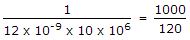# Electronics and Communication Engineering - Digital Electronics

21.

An AND gate has two inputs A and B and one inhibit input 3, Output is 1 if

 A. A = 1, B = 1, S = 1 B. A = 1, B = 1, S = 0 C. A = 1, B = 0, S = 1 D. A = 1, B = 0, S = 0

Explanation:

All AND inputs must be 1 and inhibit 0 for output to be 1.

22.

The greatest negative number which can be stored is 8 bit computer using 2's complement arithmetic is

 A. - 256 B. - 128 C. - 255 D. - 127

Explanation:

The largest negative number is 1000 0000 = -128.

23.

A JK flip flop has tpd= 12 ns. The largest modulus of a ripple counter using these flip flops and operating at 10 MHz is

 A. 16 B. 64 C. 128 D. 256

Explanation:

Number of flip-flops == 8.333 say 8

Modulus = 28 = 256.

24.

The basic storage element in a digital system is

 A. flip flop B. counter C. multiplexer D. encoder

Explanation:

Storing can be done only in memory and flip-flop is a memory element.

25.

In a ripple counter,

 A. whenever a flip flop sets to 1, the next higher FF toggles B. whenever a flip flop sets to 0, the next higher FF remains unchanged C. whenever a flip flop sets to 1, the next higher FF faces race condition D. whenever a flip flop sets to 0, the next higher FF faces race condition